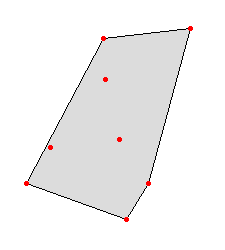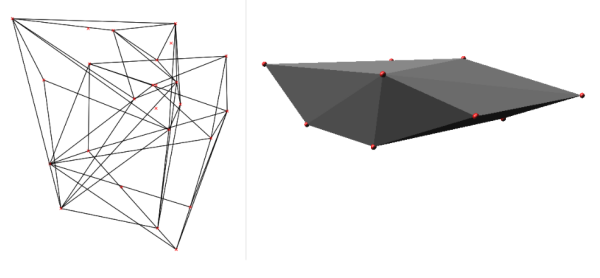## NAME

v.hull - Produces a 2D/3D convex hull for a given vector map.

## KEYWORDS

vector, geometry, 3D

## SYNOPSIS

v.hull
v.hull --help
v.hull [-rf] input=name [layer=string] output=name [cats=range] [where=sql_query] [--overwrite] [--help] [--verbose] [--quiet] [--ui]

### Flags:

-r
Limit to current region
-f
Create a 'flat' 2D hull even if the input is 3D points
--overwrite
Allow output files to overwrite existing files
--help
Print usage summary
--verbose
Verbose module output
--quiet
Quiet module output
--ui
Force launching GUI dialog

### Parameters:

input=name [required]
Name of input vector map
Or data source for direct OGR access
layer=string
Layer number or name ('-1' for all layers)
A single vector map can be connected to multiple database tables. This number determines which table to use. When used with direct OGR access this is the layer name.
Default: -1
output=name [required]
Name for output vector map
cats=range
Category values
Example: 1,3,7-9,13
where=sql_query
WHERE conditions of SQL statement without 'where' keyword
Example: income < 1000 and population >= 10000

## DESCRIPTION

v.hull computes the convex hull of a vector map and outputs the convex hull polygon as a vector area map. The convex hull, or convex envelope, for an object or a set of objects is the minimal convex set containing the given objects. This module creates a vector polygon containing all vector points or lines of the input map.

In the case of 3D input points, the hull will be a 3D hull as well, unless the user specifies the -f flag. The 3D hull will be composed of triangular faces.Fig: Convex hull polygon created with v.hull

## NOTES

In order to use the where option the layer option must be set to a positive number (the default is '-1' for 'all layers'), otherwise all points from all layers will be used and the cats and where options will be ignored.

## EXAMPLE

Example of v.hull 3D output (using two random 3D point clouds, North Carolina sample data set):
```g.region rural_1m -p
r.mapcalc "zero = 0"
v.random -z output=random3d_a n=10 zmin=0 zmax=200
v.random -z output=random3d_b n=15 zmin=400 zmax=600
v.hull input=random3d_a output=random3d_a_hull
v.hull input=random3d_b output=random3d_b_hull

d.mon wx0
d.vect random3d_a_hull
d.vect random3d_a color=red

d.vect random3d_b_hull
d.vect random3d_b color=red

# 3D view in wxGUI (g.gui)
```Fig: Convex hull in 3D from 3D points created with v.hull

## REFERENCES

• M. de Berg, M. van Kreveld, M. Overmars, O. Schwarzkopf, (2000). Computational geometry, chapter 1.1, 2-8.
• J. O'Rourke, (1998). Computational Geometry in C (Second Edition), chapter 4.

v.delaunay

## AUTHOR

Andrea Aime, Modena, Italy
Markus Neteler, ITC-irst (update to 5.7)
Benjamin Ducke, CAU Kiel (3D hull support)
Martin Landa, CTU in Prague, Czech Republic (vector lines support)

## SOURCE CODE

Available at: v.hull source code (history)

© 2003-2020 GRASS Development Team, GRASS GIS 7.8.4dev Reference Manual